Next: References Up: Sequence Comparison: Some Theory Previous: Some theory

# Some practice

In this section we restrict ourselves to some practical aspects of file comparison utilities, focusing especially on the UNIX command diff.

A file comparison utility should determine the differences between two text files. But what is the difference of two text files? Indeed, our intuitive notion of such a difference is an elusive concept and it is difficult to define it. To cope with this it became common practice to consider entire lines as indivisible objects. Then it seems that the best results are obtained if one finds a longest common subsequence of lines and then anything not in this lcs is declared a difference.

The first (and still the best) file comparison utility was included in UNIX around 1976. Since then file comparison became a standard tool and with the proliferation of microcomputers many programs turned up. These are usually called diff, but most of them do not determine a true lcs; consequently they easily missynchronize. On the other hand, some of them are very fast and work well for many pairs of (real text) files. Many of these programs are based on .

One aspect of the file comparison programs for microcomputers is worth mentioning: their output is sometimes more suggestive (for a human) of the differences than the output of the original diff. Indeed, a good way of pinpoiting the differences seems to be a simultaneous listing of both files, indicating whether each line is common to both or exclusive to one of them. Long blocks of lines in the same class might be abbreviated by showing only their first and last lines. In contrast, the output of diff is thoroughly influenced by the intricacies of machine transformation of one file in another and this restricts, in our opinion, its potential as a tool for remembering or discovering the changes during the evolution of a file.

The algorithm actually used by diff is described by Hunt and McIlroy in ; its basic idea is attributed to unpublished work of H. S. Stone who generalized ansolution of the most important particular case (the restriction of the problem to permutations) by T. G. Szymanski . The resulting algorithm is very similar to the one in ; it is also described in .

The first practical concession of diff is that it hashes the lines of the files. This is handy because it reduces significantly the volume of information to deal with. On the other hand, the hashing might introduce false matches caused by collisions; these are detected during the last phase when the computed lcs is checked in the files themselves. If false matches occur the corresponding lines are considered as differences. Consequently, it might happen that the reported common subsequence of lines is not a longest one. These events seems to be very rare in practice and the advantages of hashing greatly outweight its shortcomings.

The key concept in the algorithm is that of a k-candidate. Returning to our notations in the previous section, a k-candidate is a pair of positions (i,j) such that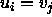and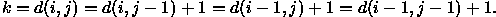It follows that every lcs ofand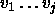is the concatenation of an lcs of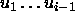and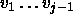with the letter. The set of k-candidates in Figure 1 is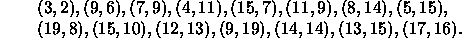The basic strategy of the algorithm is to compute the set of all k-candidates and then collect an lcs from these (such an lcs clearly exists). The computation is done by performing a binary search, in a vector of at most n components, for certain matches, that is to say, pairs (i,j) for which. Thus, r being the total number of matches this part of the algorithm takes time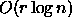(we assume throughout that both input sequences have length n). The overall worst case time complexity of the algorithm is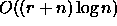and its space requirements are O(q+n), where q is the total number of k-candidates encountered.

A key question is to investigate the total number q of candidates for particular pairs of sequences. This is interesting because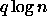and q are lower bounds for the computing time and for the space requirements, once the present strategy is adopted. Unfortunately, there are pairs, such asand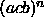or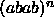and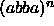for which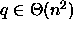. Consequently, the derived upper bound can be obtained and the complexity of the algorithm is indeed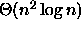, that is the worst case behavior is even worse than that of the folklore algorithm.

The great advantage of this algorithm is that in the case of permutations the number r of matches is at most n, hence the algorthm works in time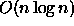. In actual practice the behavior of the algorithm is somewhere between these two bounds. Fortunately, most lines of true text files are either unique, or occur few times; hence, in practice this algorithm is definitely sub-quadratic! And this is why the algorithm works well even for long files (tens of tousands of lines).

One shortcoming of the algorithm of diff is that for families of pairs of sequences with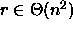the running time iseven if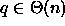. There is at least one such family of files which occur in practice and for which diff behaves badly. These are files with many occurrences of one same line, say one fourth of the lines are blank. The easiest misbehavior of diff can be obtained by running it on sequences of the formand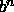.

Another shortcoming is that the computing time might depend on the order of specification of the sequences. Thus, computing the diff of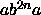andtakes much longer than the diff ofand. The fact that the difference is not a symmetrical function does not justify this behavior because what dominates the running time is the computation of an lcs and an lcs does not depend on the order of the sequences.

Both these shortcomings disappear in an interesting variant discovered recently by Apostolico in ; see also . This variant has time complexityinstead of. This is sufficient to guarantee anbehavior, instead of, for files with only one frequent line, such as the example given above. However, the gain is obtained at the expense of complicated data structures, such as balanced binary search trees, and it is not clear whether the overhead of (always) using these structures is worth the time economy which is more accentuate only for special cases. Some experimentation might throw interesting light on this question.

A family which seems to defeat every known algorithm is given by pairs of random sequences over two letters. These seem to be the real ``black sheeps'' for sequence comparison; our luck is that they do not occur in practice very frequently. Or, do they? For instance, files that have many occurrences of two different lines in interlaced positions tend to behave as random sequences over two letters. These cases might arise in practice if we have blank lines with different indentations or two lines which occur frequently, such as the pairs begin and end.

Altogether, in spite of the excellence of diff, there seems to be ample space for a substantially better algorithm, if only it could be found! But we are hopeful that the proliferation of potentially equivalent quadratic algorithms is a sign that the ultimate word was not yet said.

ACKNOWLEDGEMENTS. The author thanks Christian Choffrut for inviting him to Rouen where this work began and Dominique Perrin for insisting that this survey be presented at the 1987 Ecole de Printemps at Ile d'Oléron. I also wish to thank Alair Pereira do Lago who helped with the programming tasks and with whom I maintained many interesting and helpful discussions on the subject of this paper.Next: References Up: Sequence Comparison: Some Theory Previous: Some theory

Imre Simon
Wed May 28 19:09:09 EST 1997